# An introduction to econullnetr: null model analysis for ecological networks

### Introduction

Networks of species interactions are regularly plotted and analysed in ecology. Many of these networks are bipartite, such as pollinators and flowers, seed dispersers and plants, and parasitoids and their hosts, whilst others are more complex, such as food webs with several trophic levels. Null models can help to analyse these networks, looking at such characteristics as which species (nodes) interact with one another, the strength of individual interactions (link strengths) and the role of individual species in the network (e.g. specialist v. generalist). In particular, null models can distinguish features of the network that cannot be explained by ‘neutral’ mechanisms, such as the relative abundance of different organisms or sampling effects.

A range of R packages is available for network analysis and the econullnetr package complements them. This document provides an illustrated introduction to how to use the package and how it can be combined with other packages. For simplicity, terms are used in the broadest sense: nodes in the network are referred to as ‘species’, although in reality they could represent different taxonomic levels or other entities such as functional groups; ‘individuals’ are the individual organisms that were sampled, with each species represented by one or (usually) more individuals. Species are referred to as ‘consumers’ or ‘resources’, with the former term used when selecting which species (resources) to interact with: whilst often this involves actual consumption (e.g. predation), this need not be the case e.g. in a social network.

The null model used in econullnetr was developed in the context of testing for prey preferences by predators (Agusti et al. 2003; King et al. 2010; Davey et al. 2013). Underlying it is the hypothesis that consumers show no active preferences, such that they will ‘consume’ resources in proportion to the relative abundance of those resources (i.e. if Resource A is twice as abundant as Resource B, they will interact twice as often with A than B; Vaughan et al., in press). By holding basic characteristics of the observed data constant in the null model (e.g. number of species and number of individuals of each species), the null model represents the network patterns that could be observed, given the limitations of the observed data, in the absence of any resource selection. Differences from the null model at the level of individual interaction strengths, species-level metrics or network-level metrics, indicates the presence of resource selection.

This document provides a brief introduction to the econullnetr package, followed by three examples using data sets from the package to illustrate a range of functionality and how to combine econullnetr with two other network packages: igraph (Csardi and Nepusz 2006) and cheddar (Hudson et al., 2013). For more details of the null model, see Agusti et al., (2003) and Vaughan et al. (in press), and the individual help files for more information about the example data sets and individual functions.

### Basic summary of the package

econullnetr comprises six functions for running null models, plotting the outputs, performing a range of statistical analyses and exporting the outputs in a form that can be imported into other network packages (Table 1). The two dedicated functions for bipartite networks (bipartite_stats and plot_bipartite) are wrappers for functions in the bipartite package (Dormann et al. 2008, 2009), accessing its extensive set of tools for plotting and analysing networks.

Table 1. List of functions in econullnetr.

Name Description
generate_null_net Specify and run the null model.
test_interactions Compare observed interaction strengths to those generated
by the null model with user-defined confidence limits and
standardised effect sizes
plot_preferences Plot observed and modelled interaction strengths for
individual consumer species
bipartite_stats Compare network metrics between the observed and null
networks (bipartite networks only). A wrapper for the
bipartite package’s networklevel, grouplevel and
specieslevel functions (Dormann et al., 2008, 2009).
plot_bipartite Plot bipartite networks, calling the bipartite package’s
plotweb function (Dormann et al., 2008)
generate_edgelist Export the null model results in a standard output that
is compatible with other network analysis packages in R

### Data types

econullnetr has been designed for situations where two things are available: i) data describing the interactions between each individual consumer and the set of resources available to it (cf. already summarised at the species (node) level in an interaction matrix), and ii) independent estimates of the density of the different resources e.g. floral abundance or the density of potential prey species.

Consumer data may be collected in a range of different ways, and econullnetr has been designed to handle four main types (Vaughan et al., in press; see the generate_null_net help file for full details):

1. A single interaction observed for each individual (e.g. a pollinator sampled on the flower where it was observed or the seed species carried by an ant)
2. A list of species with which each individual interacted (e.g. a list of prey species found in an individual’s gut, but with no information about their relative abundance)
3. Counts of the number of individuals of each resource species an individual interacted with (e.g. a count of individuals of each prey species in a predator’s gut)
4. Proportions of an individual consumer’s total interactions that relate to each resource (e.g. proportion of the gut contents contributed by each prey species or proportions of pollen grains from each flower species on a pollinator’s body in a pollen transfer network)

Classes 1–2 are examples of nominal data at the level of individual consumers, whilst 3–4 are quantitative individual-level data. In all cases, the data from each individual are subsequently summarised at the species level, resulting in quantitative data: typically frequencies of interactions based on classes one and two, and mean numbers eaten or proportions for classes three and four.

### Example data sets

econullnetr includes three data sets to illustrate a range of functionality (see the individual help files for full details):

1. Silene. One of the bipartite flower visitation networks from Gibson et al., (2006), notable for the presence of small-flowered catchfly Silene gallica, a rare arable weed species in the UK. The network comprises five plant species and 128 flower visitors of 31 species.
2. WelshStreams. Part of the macroinvertebrate food web from upland streams in south Wales, UK (Pearson 2015). The network focuses on two abundant predators (Rhyacophila dorsalis and Dinocras cephalotes), with 85 individuals and 16 potential prey. Distinguished from a bipartite network by intra-guild predation between the two predators.
3. Broadstone. Part of the highly-resolved Broadstone Stream food web (Woodward et al., 2005). The data are from August 1996, with 19 macroinvertebrate taxa, including 319 individuals of seven predatory species. A matrix of ‘forbidden links’, i.e. those which cannot occur in the network, is also provided, estimated from more extensive sampling of Broadstone Stream.

### Example 1. Analysis of a bipartite network (Silene)

The Silene data illustrate a typical bipartite food web. The following workflow runs the null model and then analyses the results at the level of individual links, revealing pollinator preferences, before calculating network- and species-level statistics via the bipartite package (Dormann et al. 2008, 2009).

Start by loading up the data and running the null model:

library(econullnetr)

set.seed(1234) # To create a reproducible example
sil.null <- generate_null_net(Silene[, 2:7], Silene.plants[, 2:6], sims = 100,
data.type = "names", summary.type = "sum",
c.samples = Silene[, 1],
r.samples = Silene.plants[, 1])

The silene data were collected over 11 field visits, and so the vectors c.samples and r.samples are included to specify which visit each pollinator and accompanying floral abundance data were collected on (identical vist codes, in this case V1 to V11, must be used in the two vectors). This ensures that the null model will relate pollinators to the resources available at the time when they were collected, before combining the data from the 11 visits at the end of each iteration of the model. The visit codes are in the first column of both the pollinator and flower data sets. The data from individual pollinators is nominal, indicating the flower species upon which the pollinator was found: hence data.type = "names".

To see which links in the network are not consistent with the null model, providing evidence for flower choice, the test_interactions function is used. A warning message will appear, highlighting the importance of being aware of Type I errors, due to the large number of potential links in the network (hence a large number of individual significance tests). The results should be interpreted with caution and potentially a false discovery rate correction applied (e.g. Gotelli and Ulrich 2010):

sil.links <- test_interactions(sil.null, signif.level = 0.95)
## Warning in test_interactions(sil.null, signif.level = 0.95): Be careful of Type I errors due to the
## large number of tests

The results are a data frame with 155 rows (all possible interactions between 31 flower visitor species and five plants). To illustrate the results, show 12 rows of the table:

sil.links[40:51, ]
##               Consumer             Resource Observed Null Lower.95.CL Upper.95.CL     Test
## 40  Eristalis.pertinax       Silene.gallica        0 0.39           0       2.000       ns
## 41     Eristalis.tenax Achillea.millefolium        7 3.45           1       6.525 Stronger
## 42     Eristalis.tenax   Hypericum.pulchrum        0 6.57           3      11.525   Weaker
## 43     Eristalis.tenax       Papaver.rhoeas        0 0.39           0       2.000       ns
## 44     Eristalis.tenax     Senecio.jacobaea       16 8.68           5      12.000 Stronger
## 45     Eristalis.tenax       Silene.gallica        0 3.91           1       7.525   Weaker
## 46   Fannia.pallitibia Achillea.millefolium        1 0.05           0       1.000       ns
## 47   Fannia.pallitibia   Hypericum.pulchrum        0 0.33           0       1.000       ns
## 48   Fannia.pallitibia       Papaver.rhoeas        0 0.04           0       1.000       ns
## 49   Fannia.pallitibia     Senecio.jacobaea        0 0.40           0       1.000       ns
## 50   Fannia.pallitibia       Silene.gallica        0 0.18           0       1.000       ns
## 51 Helophilus.pendulus Achillea.millefolium        0 0.08           0       1.000       ns
##           SES
## 40 -0.6312389
## 41  2.2130379
## 42 -2.9103691
## 43 -0.6882353
## 44  3.5892739
## 45 -2.3077564
## 46  4.3370497
## 47 -0.6982922
## 48 -0.2031010
## 49 -0.8124038
## 50 -0.4661728
## 51 -0.2934058

The columns represent the consumer and resource species, followed by the observed link ‘strength’ (in this case the number of times an interaction was observed in the data), the mean link strength from the iterations of the null model, and the confidence limits for the specified level of statistical significance. The penultimate column indicates whether the observed interaction is significantly stronger or weaker than expected under the null model (i.e. whether the observed value is > upper confidence limit or < lower confidence limit, respectively), or consistent with the null model (ns; with the observed value falling within the confidence interval). The final column is the standardised effect size (SES), presenting the difference between the observed and null model values in a format that can be compared more generally (Gotelli and McCabe 2002): although care is required in using SES to compare among networks (Song et al. 2017).

In this example, the hoverfly Eristalis tenax was expected to visit both Hypericum pulchrum and Silene gallica several times (6.6 and 3.9 times respectively), but was never observed to do so, suggesting that it tended to avoid these two flowers. Conversely, Senecio jacobaea was visited nearly twice as often as expected (16 times versus 8.7), indicative of a preference for it. Visits to the other two flower species were consistent with the null model. In total, 12 out of the 155 interactions differed significantly from the null model:

sum(sil.links$Test == "Weaker" | sil.links$Test == "Stronger")
##  12

The interaction-level results can be viewed in two ways. The first is using plot_bipartite, which is useful for showing where the stronger/weaker links occur in the network. This function is a wrapper for bipartite’s plotweb function (Dormann et al. 2008), and most of its arguments can be used to customise the output: plot_bipartite simply colour codes the links to reflect the null modelling results:

# Calculate the mean abundance of each plant species across all samples to
#   use in the bipartite plot
mean.abunds <- colMeans(Silene.plants[, 2:6])

plot_bipartite(sil.null, signif.level = 0.95,
edge.cols = c("#67a9cf", "#F7F7F7", "#ef8a62"),
low.abun = mean.abunds, abuns.type = "independent",
low.abun.col = "black", high.abun.col = "black",
high.lablength = 5, low.lablength = 5)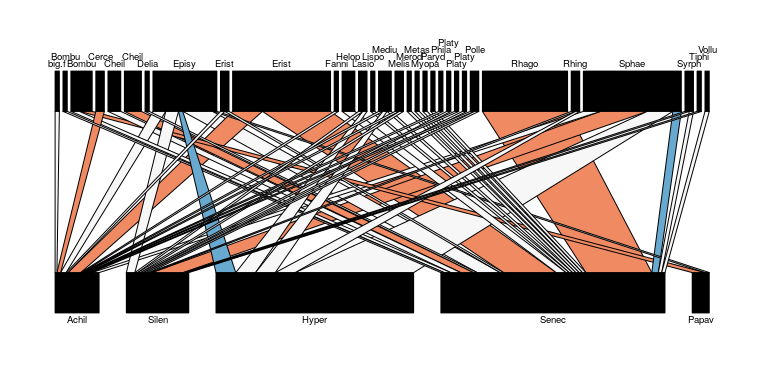There is clear evidence for Senecio being favoured by several flower visitors (e.g. the hoverfly Eristalis tenax ‘Erist’ and beetle Rhagonycha fulva (‘Rhago’)), but also apparent avoidance by the hoverfly Sphaerophoria scripta (‘Sphae’). The limitation of this plot is that the relative strength of the observed and modelled interactions cannot easily be shown. For this, a second plotting function is provided plot_preferences. This displays the observed interaction strengths, modelled confidence limits and whether interactions are stronger or weaker than expected for one consumer species at a time. For the hoverfly Sphaerophoria scripta:

plot_preferences(sil.null, "Sphaerophoria.scripta", signif.level = 0.95,
type = "counts", xlab = "Number of visits", p.cex = 2,
l.cex = 1, lwd = 2, font = 3)
## Warning in test_interactions(nullnet, signif.level = signif.level): Be careful of Type I errors due
## to the large number of tests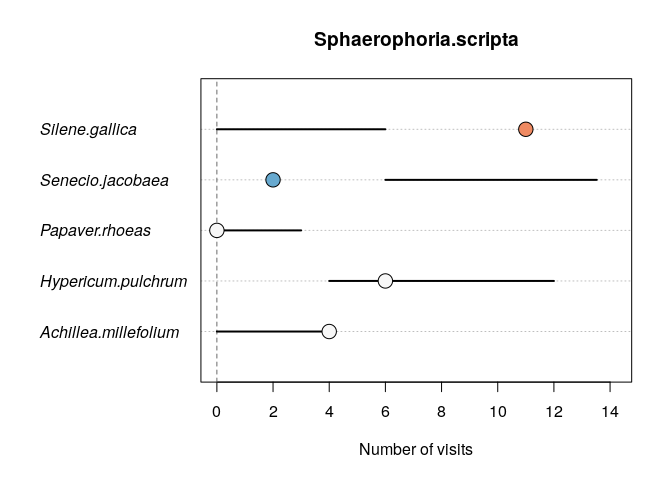The bars represent the 95% confidence limits from the null model, which overlap for three of the plant species (A. millefolium, H. pulchrum and P. rhoeas), indicating that the observed frequency falls within the range of values expected under the null model. A near four-fold preference for Silene was evident, apparently at the expense of Senecio, which was only visited around 20% as often as expected.

Preferences for individual resources may in turn be evident at the level of species (e.g. whether a consumer species is a specialist) and in the structure of the whole network. The bipartite_stats function calls one of the bipartite package’s specieslevel, grouplevel or networklevel functions to calculate statistics for the observed network, and across the iterations of the null model, to assess any differences. A simple illustration at the network-level:

net.stats <- bipartite_stats(sil.null, index.type = "networklevel",
indices = c("linkage density",
"weighted connectance", "weighted nestedness",
"interaction evenness"), intereven = "sum")
net.stats
##                       Observed      Null  Lower.CL  Upper.CL  Test       SES
## weighted nestedness  0.5078259 0.5664086 0.3328021 0.7410961    ns -0.555432
## linkage density      5.0960242 6.6976881 6.1663787 7.2166387 Lower -5.670941
## weighted connectance 0.1415562 0.1863083 0.1712883 0.2004622 Lower -5.717131
## interaction evenness 0.8489881 0.8973740 0.8822482 0.9143993 Lower -5.694904

Linkage density, connectance and evenness are all lower than expected under the null model, consistent with flower visitors showing preferences for the different flower species. Various mechanisms could be involved, including the accessibility of nectar based on flower morphology and the quantity of nectar different flowers produce. Nestedness was consistent with the null model, suggesting that the choices that the flower visitors made did not collectively increase or decrease nestedness over that generated by a neutral mechanism (e.g. differences in the abundance of different insects and flower species through time).

### Example 2. Analysis of part of a food web, using igraph for plotting

The WelshStreams data set is not bipartite due to intra-guild predation between the two predator species, meaning that a different approach is needed to plot the data. This example shows how econullnetr can be combined with igraph (Csardi & Nepusz 2006) for plotting. The example includes a table specifying ‘forbidden links’ which are excluded from the null model. As the original data were collected using molecular screening of gut contents to identify prey DNA, they cannot distinguish host DNA from cannibalism, and so two forbidden links are specified to rule out cannibalism from the null model so that it is directly comparable to the observed data.

set.seed(1234)
stream.1 <- generate_null_net(WelshStreams[, 2:18], WelshStreams.prey[, 2:17],
sims = 100, data.type = "names",
summary.type = "sum",
c.samples = WelshStreams[,1],
r.samples = WelshStreams.prey[,1],
r.weights = WelshStreams.fl)
## Warning in generate_null_net(WelshStreams[, 2:18], WelshStreams.prey[, 2:17], : One or more instances detected where a consumer interacted with a
##        resource that has zero abundance in 'resources'

A warning message appears to highlight that there are two instances where the predators consumed a taxon that was not found in the kick sample for that stream: one stream each for Asellidae and Philopotamus. For simplicity, these will be ignored here, but in other situations it may be desirable to take action e.g. removing these taxa altogether or adding a small non-zero constant to the abundance data so that they could be sampled in the null model.

Preference plots are created for the two predators, using the data frame WelshStreams.order to set the order in which bars are plotted: this is the taxonomic order according to the Centre for Ecology and Hydrology’s coded macroinvertebrate list.

par(mfrow = c(1, 2))
plot_preferences(stream.1, "Rhyacophila", signif.level = 0.95, type = "counts",
xlab = "Num. of prey detections", res.order =
WelshStreams.order, p.cex = 1.5, l.cex = 0.9, lwd = 2)
plot_preferences(stream.1, "Dinocras", signif.level = 0.95, type = "counts",
xlab = "Num. of prey detections", res.order =
WelshStreams.order, p.cex = 1.5, l.cex = 0.9, lwd = 2)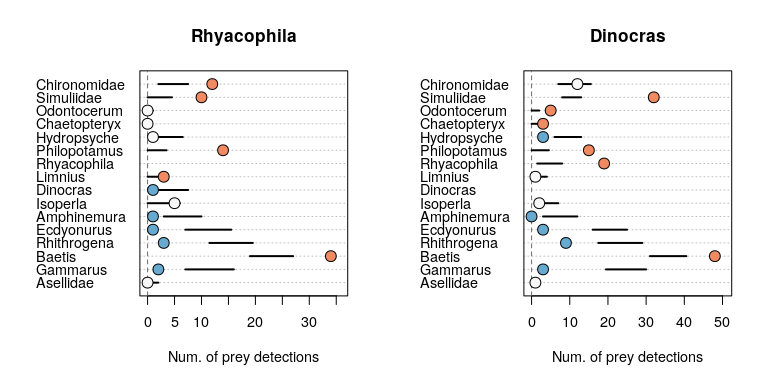The two predator species show similar overall preferences, one of the key differences being the asymmetry of the intraguild predation: the caddisfly Rhyacophila predated the stonefly Dinocras less often than expected, whilst Dinocras appeared to favour Rhyacophila.

The next stage is to export the null modelling results to igraph, using the generate_edgelist function:

export1 <- generate_edgelist(stream.1, signif.level = 0.95,
edge.cols = c("#2c7bb6", "#000000", "#d7191c"))

The edge.cols argument allows the preferred colour scheme for stronger/weaker links to be included in the data frame. A basic call to igraph to import the network is:

library(igraph)
##
## Attaching package: 'igraph'
## The following objects are masked from 'package:stats':
##
##     decompose, spectrum
## The following object is masked from 'package:base':
##
##     union
net.1 <- graph_from_edgelist(as.matrix(export1[, c("Resource", "Consumer")]),
directed = TRUE)

# Add in the null modelling results
E(net.1)$obs.str <- export1$Observed
E(net.1)$test.res <- export1$Test
E(net.1)$edge.cols <- export1$edge.col

plot(net.1, layout = layout_in_circle, edge.color = E(net.1)$edge.cols, edge.width = sqrt(E(net.1)$obs.str))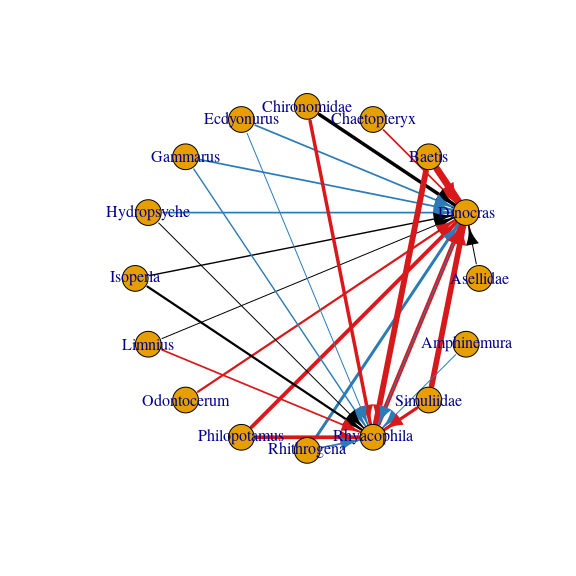Black links are consistent with the null model, whilst red links are stronger than expected and blue ones are weaker than expected.

With some additional commands it is possible to take greater control of the plot and make it more informative. Here, the position of the nodes is set manually to create a two-level bipartite-style plot, with prey taxa arranged in taxonomic order. The size of the prey nodes is proportional to their abundance:

# Create a data frame to assist with plotting
# trph.level = a simple trophic level: primary consumers = 1, predators = 2
n.names <- data.frame(species = V(net.1)$name, trph.level = c(1, 2, 1, 1, 1, 1, 1, 1, 1, 1, 1, 1, 1, 2, 1, 1)) # Calculate prey abundance across the six streams abund <- as.matrix(colSums(WelshStreams.prey[, 2:17]) ) n.names <- merge(n.names, abund, by.x = "species", by.y = "row.names", sort = FALSE) colnames(n.names) <- "Abundance" # Specify x-coordinates for the nodes, based on their order in the Centre for Ecology and Hydrology's Coded Macroinvertebrate List, positions for the labels and abbreviated names for the nodes n.names$x.coord <- c(1, 4.5, 3, 11, 14, 5, 2, 10, 7, 8, 12, 9, 4, 10.5, 13, 6)
n.names$lab.deg<-c(pi/2,-pi/2, pi/2, pi/2, pi/2, pi/2, pi/2, pi/2, pi/2, pi/2, pi/2, pi/2, pi/2, -pi/2, pi/2, pi/2) n.names$short.names <- strtrim(n.names$species, 4) n.names$short.names <- "Dinocras"
n.names$short.names <- "Rhyacophila" # Create curved edges between Dinocras & Rhyacophila so that predation in both directions can be shown clearly. E(net.1) # Confirms that edge 13 = Rhyacophila to Dinocras  ## + 1/26 edge from bb94142 (vertex names): ##  Rhyacophila->Dinocras E(net.1) # Confirms that edge 18 = Dinocras to Rhyacophila ## + 1/26 edge from bb94142 (vertex names): ##  Dinocras->Rhyacophila curve.edge <- rep(0, ecount(net.1)) curve.edge[c(13, 18)] <- 0.5 curve.arrows <- rep(0, ecount(net.1)) curve.arrows[c(13, 18)] <- 2 # Create the food web plot plot(net.1, layout = as.matrix(n.names[, c("x.coord", "trph.level")]), vertex.shape = "rectangle", vertex.size = log(n.names$Abundance),
vertex.size2 = 20, edge.curved = curve.edge, edge.arrow.mode = curve.arrows,
edge.color = E(net.1)$edge.cols, edge.width = sqrt(E(net.1)$obs.str),
vertex.color = "#000000", vertex.label = n.names$short.names, vertex.label.degree = n.names$lab.deg, vertex.label.family = "",
vertex.label.dist = rep(3, 16), vertex.label.cex = 0.75, asp = .4)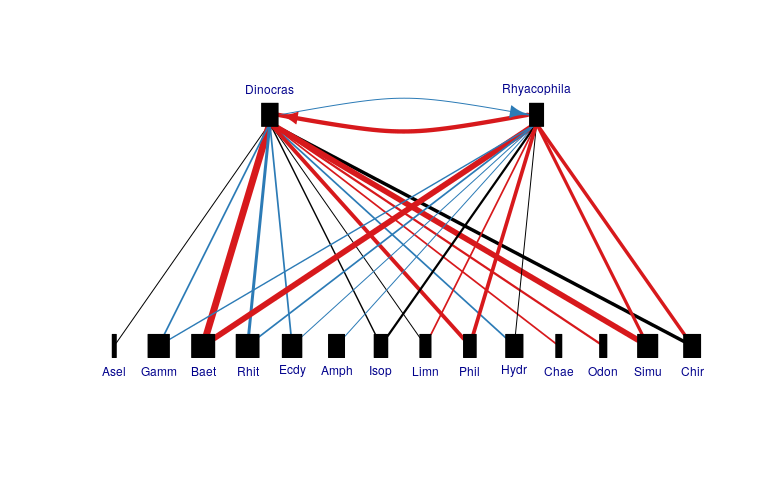This highlights prey favoured by both predators (e.g. Baetis, Simuliidae), avoided (e.g. Rhithrogena) and the asymmetry in the intra-guild predation.

### Example 3. Analysis and plotting of a food web using the cheddar package for calculating network statistics and plotting

The Broadstone Stream data represent a highly resolved freshwater food web, requiring different tools to be used with econullnetr to calculate network metrics and for plotting the network. This example uses some of the functions from the cheddar package (Hudson et al., 2013) to help to identify dietary specialisation amongst predatory species and plot prey preferences on the network. This is done by calculating the generality (Bersier et al. 2002) of the seven predator taxa’s diets using cheddar’s NodeQuantitativeDescriptors function, and comparing it to the generality estimated under the null model to indicate whether diets were more or less general than expected based on the distribution of resource abundances.

The Broadstone web is well studied, giving a good idea of the breadth of possible – and forbidden – trophic interactions, allowing more realistic food webs to be modelled. To approximate the forbidden links, the data frame Broadstone.fl uses the complete set of interactions observed across a complete year’s sampling of the web (see Woodward et al., 2005 for details). The BS.nodes data frame provides two additional variables for use with cheddar: the mean size of an individual of each taxon (taken from BroadstoneStream in cheddar) and the abundance of each taxon in the kick sample data. The first steps are to read in the data and run the null model, after which it is straightforward to create a Community object in cheddar from a econullnetr edgelist:

# The Broadstone data comprise counts of different prey taxa in each individual predator's gut; hence data.type = "counts"
set.seed(1234)
bs.null <- generate_null_net(Broadstone, Broadstone.prey, sims = 100,
data.type = "counts", summary.type = "sum",

library(cheddar)
# Create a list of properties, used for creating a cheddar 'Community' object
BS.props <- list(title = "Broadstone, August", M.units = "mg", N.units = "counts")
BS.links <- generate_edgelist(bs.null, signif.level = 0.95,
edge.cols = c("#2c7bb6", "#000000", "#d7191c"))
colnames(BS.links)[1:2] <- c("consumer", "resource") # Change to lower case to match cheddar
BS <- Community(nodes = Broadstone.nodes, properties = BS.props,
trophic.links = BS.links)

Several plotting options are available at this stage, including a traditional food web plot and plot of the food web matrix. In both cases, red and blue indicate links that are stronger or weaker respectively than expected under the null model. In the first plot, link thickness represents the observed number of prey eaten:

# Traditional multi-level food web plot
PlotWebByLevel(BS, link.colour.by = "Test", link.colour.spec = c(Stronger =
"#d7191c", ns = "#cccccc", Weaker = "#2c7bb6"), link.lwd =
log(TLPS(BS)$Observed), pch = 16, cex = 3, col = "black", highlight.nodes = NULL, show.nodes.as = "both", label.cex = 1, label.colour = "white") legend("topright", legend = c("Stronger", "ns", "Weaker"), lty = 1, lwd = 2, col = c("#d7191c", "#cccccc", "#2c7bb6"))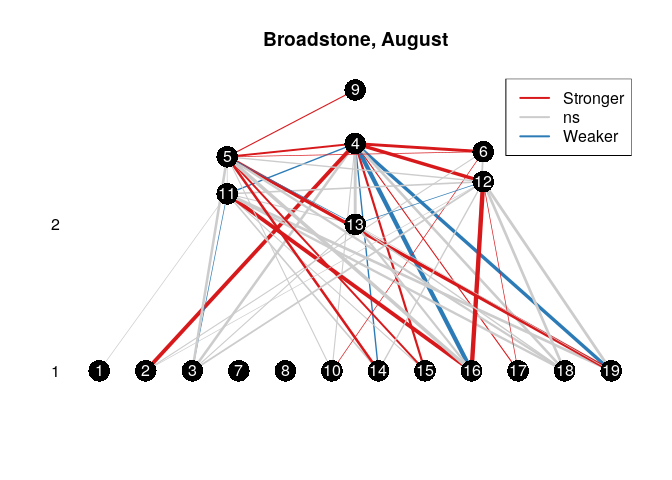# Alternative representation, plotting the predation matrix PlotPredationMatrix(BS, resource.order = "M", consumer.order = "M", pch = 15, cex = 2, colour.by = "Test", main = NULL, ylab = "", colour.spec = c(Stronger = "#d7191c", ns = "#cccccc", Weaker = "#2c7bb6")) axis(2, at = seq(1, 19), labels = strtrim(NPS(BS)$node[order(NPS(BS)$M, decreasing = TRUE)], 6), las = 1) axis(3, at = seq(1, 19), labels = strtrim(NPS(BS)$node[order(NPS(BS)$M)], 6), las = 3)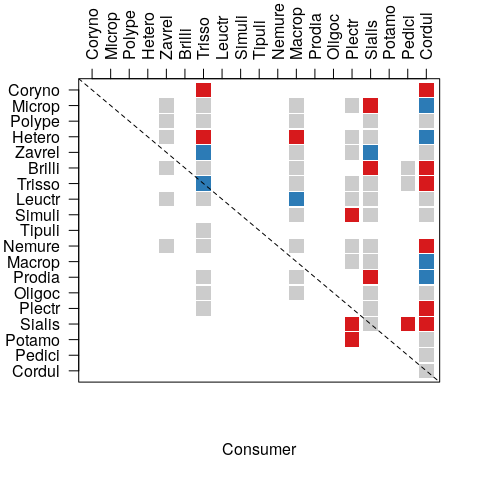A simple workflow can be created to combine econullnetr and cheddar to test for significant differences in network statistics between the observed and modelled networks: library(reshape2) for (i in 1:bs.null$n.iterations) { # Number of null model iterations
net.data <- bs.null$rand.data[bs.null$rand.data$Iteration == paste("It.", i, sep = ""), -1] net.data <- melt(net.data, id.vars = "Consumer") colnames(net.data) <- c("consumer", "resource", "Freq") BS.rand <- Community(nodes = Broadstone.nodes, properties = BS.props, trophic.links = net.data) BS.gen <- data.frame(NodeQuantitativeDescriptors(BS.rand, "Freq"))$g
ifelse(i == 1, Gen <- BS.gen, Gen <- cbind(Gen, BS.gen))
}

# Calculate the percentiles for the 95% confidence interval and calculate
#   test results. ‘Higher’, ‘Lower’, not significant ‘ns’ or not applicable
#   due to being a primary consumer(‘P.cons’)

gen.cl <- apply(Gen, 1, quantile, probs = c(.025, .975))
gen.summary <- data.frame(obs = data.frame(NodeQuantitativeDescriptors(BS,
"Observed"))$g, gen.2.5 = t(gen.cl)[, 1], gen.97.5 = t(gen.cl)[, 2]) gen.tests <- rep("ns", 19) for (i in 1:19) { if(max(gen.summary[i, ]) == 0) gen.tests[i] <- "P.cons" if(gen.summary$obs[i] > gen.summary$gen.97.5[i]) gen.tests[i] <- "Higher" if(gen.summary$obs[i] < gen.summary$gen.2.5[i]) gen.tests[i] <- "Lower" } It is then straightforward to plot the food web, using different symbols for primary consumers and predators, and colour coding the predator species according to whether their diets are more general than expected under the null model (red), more specialised (blue) or consistent with the null model (black): PlotWebByLevel(BS, link.colour.by = "Test", link.colour.spec = c(Stronger = "#d7191c", ns = "#cccccc", Weaker = "#2c7bb6"), link.lwd = log(TLPS(BS)$Observed), symbol.by = gen.tests, symbol.spec =
c(Higher = 15, P.cons = 16, ns = 15, Lower = 15), cex = 3,
colour.by = gen.tests, colour.spec = c(Higher = "#d7191c",
P.cons = "#636363", ns = "#000000", Lower = "#2c7bb6"),
highlight.nodes = NULL, main = "Generality", show.nodes.as = "both",
label.cex = 1, label.colour = "white")
legend("topright", legend = c("1", "10", "100"), lty = 1,
lwd = c(log(1), log(10), log(100)), col = 1, title = "Number eaten")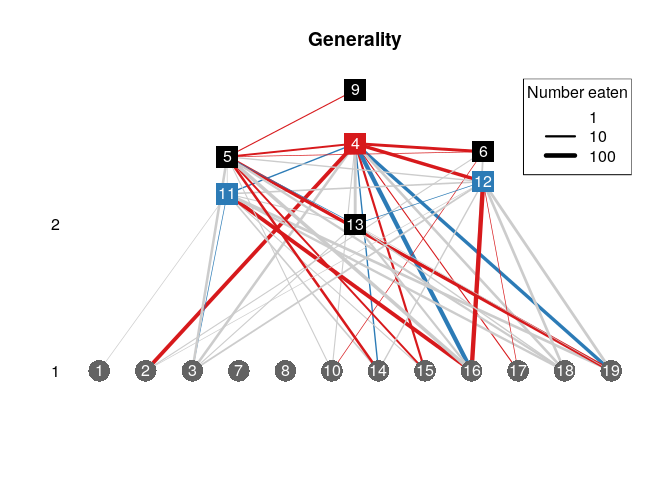In a final stage, preference plots reveal the apparent prey preferences that contribute to more specialised (Trissopelopia; node 12) or less specialised Cordulegaster (node 4) diets than expected. Prey taxa are plotted in order of increasing size on the y-axis (smallest taxon at the bottom):

par(mfrow = c(1, 2))
plot_preferences(bs.null, "Trissopelopia", signif.level = 0.95, type = "counts",
xlab = "Number of visits", p.cex = 1.5, l.cex = 0.9, lwd = 2,
res.order =  data.frame(Broadstone.nodes$node, Broadstone.nodes$M))
## Warning in test_interactions(nullnet, signif.level = signif.level): Be careful of Type I errors due
## to the large number of tests
plot_preferences(bs.null, "Cordulegaster", signif.level = 0.95, type = "counts",
xlab = "Number of visits", p.cex = 1.5, l.cex = 0.9, lwd = 2,
res.order = data.frame(Broadstone.nodes$node, Broadstone.nodes$M))
## Warning in test_interactions(nullnet, signif.level = signif.level): Be careful of Type I errors due
## to the large number of tests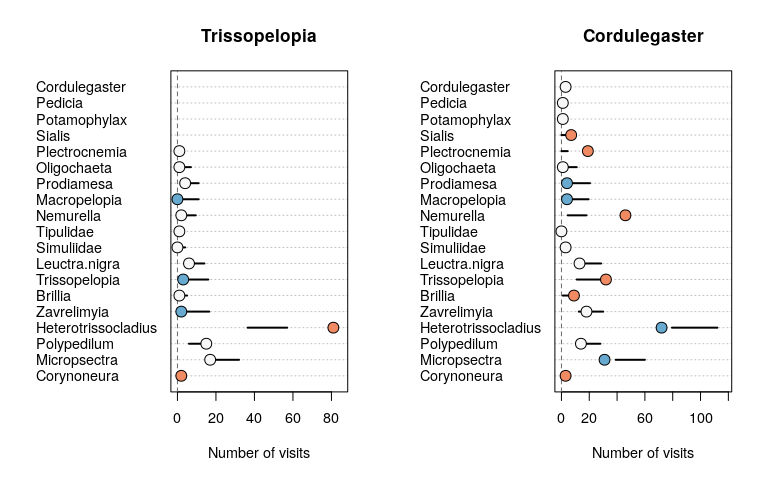Trissopelopia, a small chironomid midge is one of the smallest predators in the web, and showed strong preference for Heterotrissocladius, another small chironomid that occupies similar interstitial microhabitats. Most of the remaining taxa were consumed at rates consistent with the null model or eaten less often than expected (e.g. Zavrelimyia). Cordulegaster, by contrast, was the largest invertebrate predator in the web, limiting its access into small interstitial spaces in the stream bed. In addition, it has mouthparts that allow it to take large prey. It tended to consume smaller, abundant, interstitial taxa less often than expected and larger, less abundant taxa more often than expected, leading to its more general diet than predicted by the model.